# Quiz 16: Rent, Interest, and Profit

To find the average tax rate of each individual, we simply see what proportion of their income the tax takes away. For the first individual, he is taxed \$2,000 for an income of \$10,000, therefore, the tax iS20% of his incomeFor the second individual, who has an income of \$20,000, he pays \$3,000 in tax. We see then that the tax of \$3,000 iS15% of the second individual's income of \$20,000Finally, the third individual pays \$4,000 in taxes for \$30,000 of income. This means that he payS13.3% of his income in taxes (\$4,000/\$30,000).We see then that the tax in this economy is one that is regressive, as person 1, who had the lowest income, paid the highest tax rate (20%). Person 2 had a higher income and paid a lower tax rate of 15%. Finally, the individual with the highest income had an even lower tax rate of 13.3%. Remember that a regressive tax is simply a tax where the tax rate falls as income goes up, as we see here.
It is given that the income of individual is \$50,000. So, the tax paid on the income is calculated over each tax brackets as follows: Taxes paid on first 10,000 at the rate of 0% is \$0. Taxes paid on the earning between \$10,000 and \$20,000 at the rate of 20% is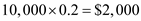. Taxes paid on the earning between \$20,000 and \$30,000 at the rate of 30% is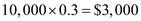. Since his earning is \$50,000 so the taxes paid on the earning from \$30,000 to \$50,000 at the rate of 40% is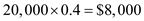. Hence, the total tax paid over the earnings of \$50,000 is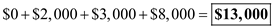. Since the rate of tax on the last dollar earned is 40%, therefore the marginal tax rate is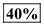. Average tax rate is calculated below:Hence, the average tax rate is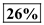. The given tax system is progressive because the successive higher income brackets are taxed at higher tax rate. In other words, the marginal tax rate as well as average tax rate for each higher successive tax slab is higher.With increase in earnings over \$30,000 the average tax rate will approximately equal to marginal tax rate.